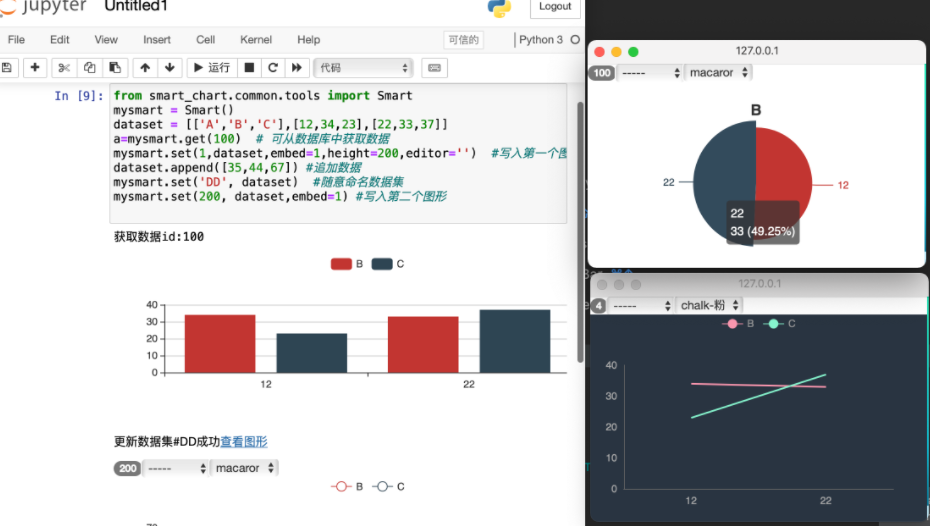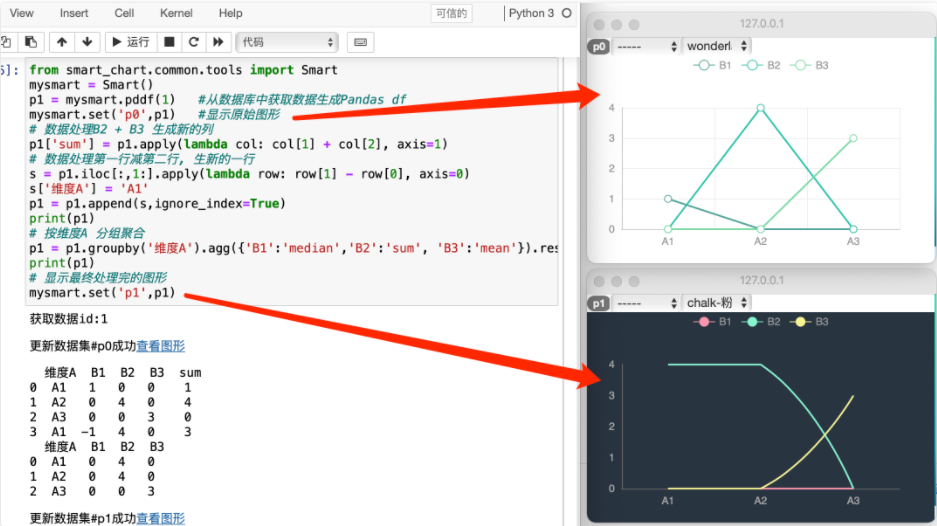Jupyter中使用指引

### 应用场景 #

Smartchart支持像pyecharts, Matplotlib 等python绘图工具一样在Jupyter中使用, 但她更加方便, 更加炫酷 和 通用化, 不仅仅是一个绘图工具, 而且是一个平台### 安装使用方法 #

``````你需要在jupyter相同的python环境中安装smartchart客户端
pip install smartchart

``````

### 快速开始 #

``````from smart_chart import Smart
Smart().set_auth('用户名', '密码',url = 'http://xxxxx')
``````

``````Smart().set_auth('用户名', '密码')
``````

``````from smart_chart import Smart
mysmart = Smart()
dataset = [['A','B','C'],[12,34,23],[22,33,37]]
#把数据写入临时数据集并显示图形
mysmart.set(1,dataset)
#随意命名临时数据集, 不一定需要smartchart中数据集已有的
mysmart.set('DD', dataset)

#从已有的数据集中获取数据(格式参考smartchart数据集)
ds1 = mysmart.get(1)
ds2 = mysmart.get('DD')
``````

### 修改图形显示 #

``````mysmart.set('barxxx', dataset)  #显示柱形图, 另外还有linexxx, piexxx
``````

``````mysmart.set('myds_1', dataset, push=1)  #参数push=1, 将实例化数据集
``````

``````#不加push, 将使用新的数据采用myds_1的图形临时显示, 而不会改变原myds_1的数据
mysmart.set('myds_1', dataset)
``````

### 显示设定参数 #

``````# width, height指定图形嵌入显示的宽高
# embed 默认不嵌入, embed=1 嵌入, embed=0 不嵌入
# editor 是否显示图形菜单, 1显示, 0不显示
# push 是否持久化数据集 push=1, 无则新建有则保存数据
# url 报表访问的url,默认是localhost

#可以全局初始化设定
mysmart = Smart(width=xx, height=xx, embed=1, editor='')
#也可以全局单独进行设定
mysmart.url = 'http://ip:8000'
mysmart.embed = 1

#也可以针对单独的一个图形设定
mysmart.set(1,dataset,embed=1,height=200,editor=0)
``````

### SmartChart与Pandas #

Smartchart的set支持直接set Pandas的dataframe对象

``````from smart_chart import Smart
import pandas as pd
mysmart = Smart()
mysmart.set('excelsample', df.sample(10))

df1 = df.groupby('province').agg({'qty':'sum'}).reset_index()
mysmart.set('ec_df1', df1, push=1)

df2 = df.groupby('c1').agg({'qty':'sum'}).reset_index()
mysmart.set('ec_df2', df2, push=1)

df4 = df.groupby('province').agg({'qty':'count'}).reset_index()
mysmart.set('ec_df4', df1, push=1)

df3 = df.groupby('c3').agg({'qty':'sum'}).reset_index()
print(df3)
df3.loc[1, 'qty'] = df3.loc[1, 'qty'] * 100
print(df3)

mysmart.set('ec_df3', df3, push=1)

#mysmart.set('pie0', df1)
#df2 = df.groupby(['province','c3']).agg({'qty':'sum'}).reset_index()
#print(df2)
#mysmart.set('ssss', df2)
#print(mysmart.get(15))
``````